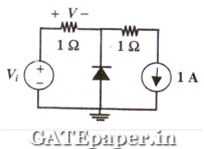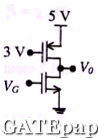### GATE 2009 ECE Video Solutions on EDC (Electronic Devices and Circuits)

1. In an N type silicon crystal at room temperature, which of the following can have a concentration of 4x1019 cm-3?
a. Silicon atoms
b. Holes
c. Dopant atoms
d. Valence electrons

2. The full forms of the abbreviations TTL and CMOS in reference to logic families are
a. Triple Transistor Logic and Chip Metal Oxide semiconductor
b. Tristate Transistor Logic and Chip Metal Oxide semiconductor
c. Transistor Transistor Logic and Complementary Metal Oxide semiconductor
d. Tristate Transistor Logic and Complementary Metal Oxide semiconductor

3. The ratio of the mobility to the diffusion coefficient in a semiconductor has the units
a. V-1
b. cm. V-1
c. V. cm-1
d. V.sec

4. In the circuit below, the diode is ideal. The voltage V is given bya. Min(Vi,1)
b. Max(Vi,1)
c. Min(-Vi,1)
d. Max(-Vi,1)

5. Consider the following two statements about the internal conditions in an N channel MOSFET operating in the active region.
S1: the inversion charge decreases from source to drain
S2: the channel potential increases from source to drain
Which of the following is correct?
a. Both are TRUE
b. Both are FALSE
c. Both are TRUE, but S2 is not a reason for S1
d. Both are TRUE, and S2 is a reason for S1.

6. Common Data Question:
Consider a silicon PN junction at room temperature having the following parameters:
Doping on the N side = 1x1017 cm-3
Depletion width on N side = 0.1 µm
Depletion width on the P side = 1 µm
Intrinsic carrier concentration = 1.4x1010 cm-3
Thermal voltage = 26 mV
Permittivity of free space = 8.85x10-14 F/cm
Dielectric constant of silicon = 12

i. The built in potential of the junction is
a. 0.70 volts
b. 0.76 volts
c. 0.82 volts
d. Cannot be estimated from given data

ii. The peak electric field in the device is
a. 0.15 Mvolts/cm, directed from P region to N region
b. 0.15 Mvolts/cm, directed from N region to P region
c. 1.80 Mvolts/cm, directed from P region to N region
d. 1.80 Mvolts/cm, directed from N region to P region

Consider the CMOS circuit shown, where the gate voltage VG of the N channel MOSFET is increased from zero, while the gate voltage of the P channel MOSFET is kept constant at 3 volts. Assume that, for both transistors, the magnitude of the threshold voltage is 1 volts and the product of the transconductance parameter and the (W/L) ratio i.e. the quantity µcox(W/L) is 1 mA/V2.i. For small increase in VG beyond 1 volt, which of the following gives the correct description of the region of operation of each MOSFET?
a. Both are in saturation region
b. Both are in triode region
c. NMOS is in triode region and PMOS is in saturation region
d. NMOS is in saturation region and PMOS is in triode region

ii. Estimate the output voltage, Vo for VG = 1.5 volts. (Hint: use the appropriate current voltage equation for each MOSFET, based on the answer to above question)